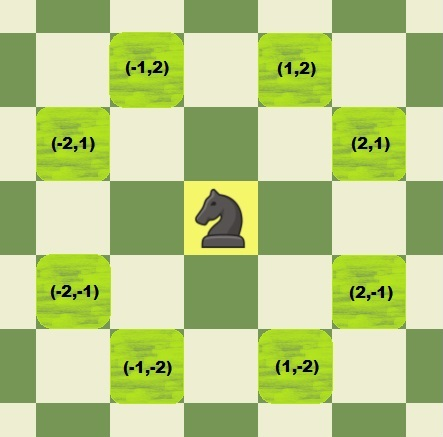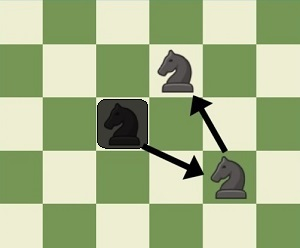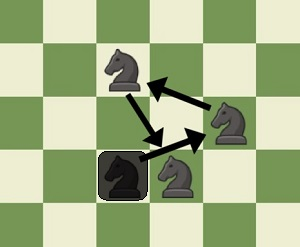Problem title
Difficulty
Avg time to solve

Get DFS Path
Easy
15 mins
Minimum Knight Moves
Hard
--
Easy
--
Check Equations
Moderate
--
Best Time to Buy and Sell Stock with Transaction Fee
Moderate
--
Duplicate Characters
Easy
25 mins
Make It Palindrome
Easy
25 mins
Maximum Points
Easy
10 mins
Partition Array for Maximum Sum
Moderate
--
Remove character
Easy
--5

# Minimum Knight Moves

Difficulty: HARD

Problem Statement

#### For example :

``````As depicted in the photo below, the knight currently at (0, 0) can move to any of the 8 positions: (1, 2), (2, 1), (2, -1), (1, -2), (-1, -2), (-2, -1), (-2, 1), (-1, 2).
``````##### Example :
``````If X = 1 and Y = -1, then we need to find out the minimum number of steps to move the knight from (0, 0) to (1, -1).

We need at least 2 steps to move the knight to the desired position.

First move: (0, 0) -> (2, 1)

Second move: (2,1) -> (1, -1)

Here we can see that there are many ways, but we need at least 2 steps. Therefore we will return the value 2.
``````
##### Input Format :
``````The first line contains a single integer ‘T’ denoting the number of test cases, then each test case follows:

The first and only line of each test case contains two integers ‘X’ and ‘Y’, denoting the x-coordinate and y-coordinate of the final position of the knight.
``````
##### Output Format :
``````For each test case, print the minimum number of steps needed.

Output for each test case will be printed in a separate line.
``````
##### Note :
``````You are not required to print anything; it has already been taken care of. Just implement the function.
``````
##### Constraints :
``````1 <= T <= 10
-100 <= X, Y <= 100

Time limit: 1 sec
``````
##### Sample Input 1 :
``````2
1 1
1 0
``````
##### Sample Output 1 :
``````2
3
``````
##### Explanation For Sample Input 1 :
``````For test case 1 :
(0, 0)  to (2, -1) to (1,1), therefore 2 steps are required. The other possible way is (0, 0) to (-1, 2) to (1, 1), but we require at least 2 steps to move from (0,0) to (1,-1).

Hence return value 2. Refer the image for better understanding:
````````````For test case 2 :
(0, 0) to (2, 1) to (0, 2) to (1, 0), therefore 3 steps are required. Refer the image for better understanding:
``````##### Sample Input 2 :
``````2
12 5
5 12
``````
##### Sample Output 2 :
``````7
7
``````Console